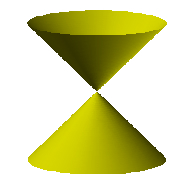index: click on a letter A B C D E F G H I J K L M N O P Q R S T U V W X Y Z A to Z index index: subject areas numbers & symbols sets, logic, proofs geometry algebra trigonometry advanced algebra & pre-calculus calculus advanced topics probability & statistics real world applications multimedia entrieswww.mathwords.com about mathwords website feedback

 Double Cone A geometric figure made up of two right circular cones placed apex to apex as shown below. Typically a double cone is considered to extend infinitely far in both directions, especially when working with conic sections and degenerate conic sections. Note: The graph of the equation z2 = x2 + y2 is a standard way to represent a double cone. That is the equation for the image below.Double Cone